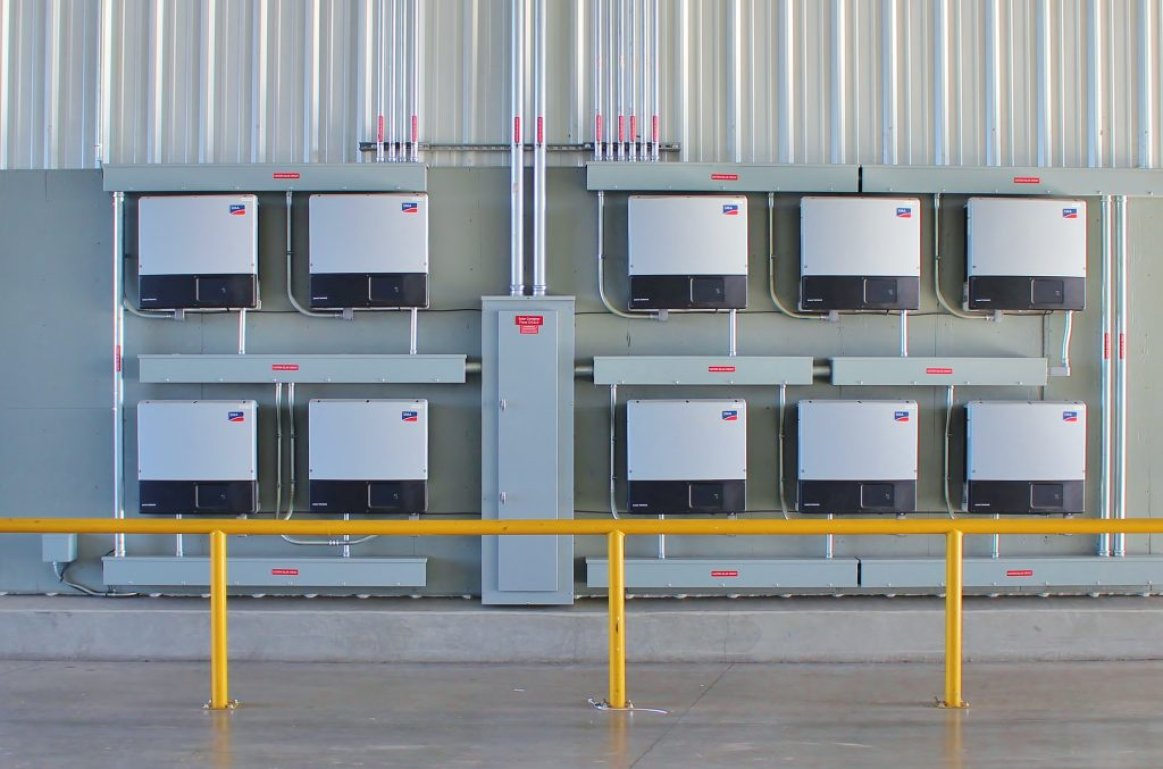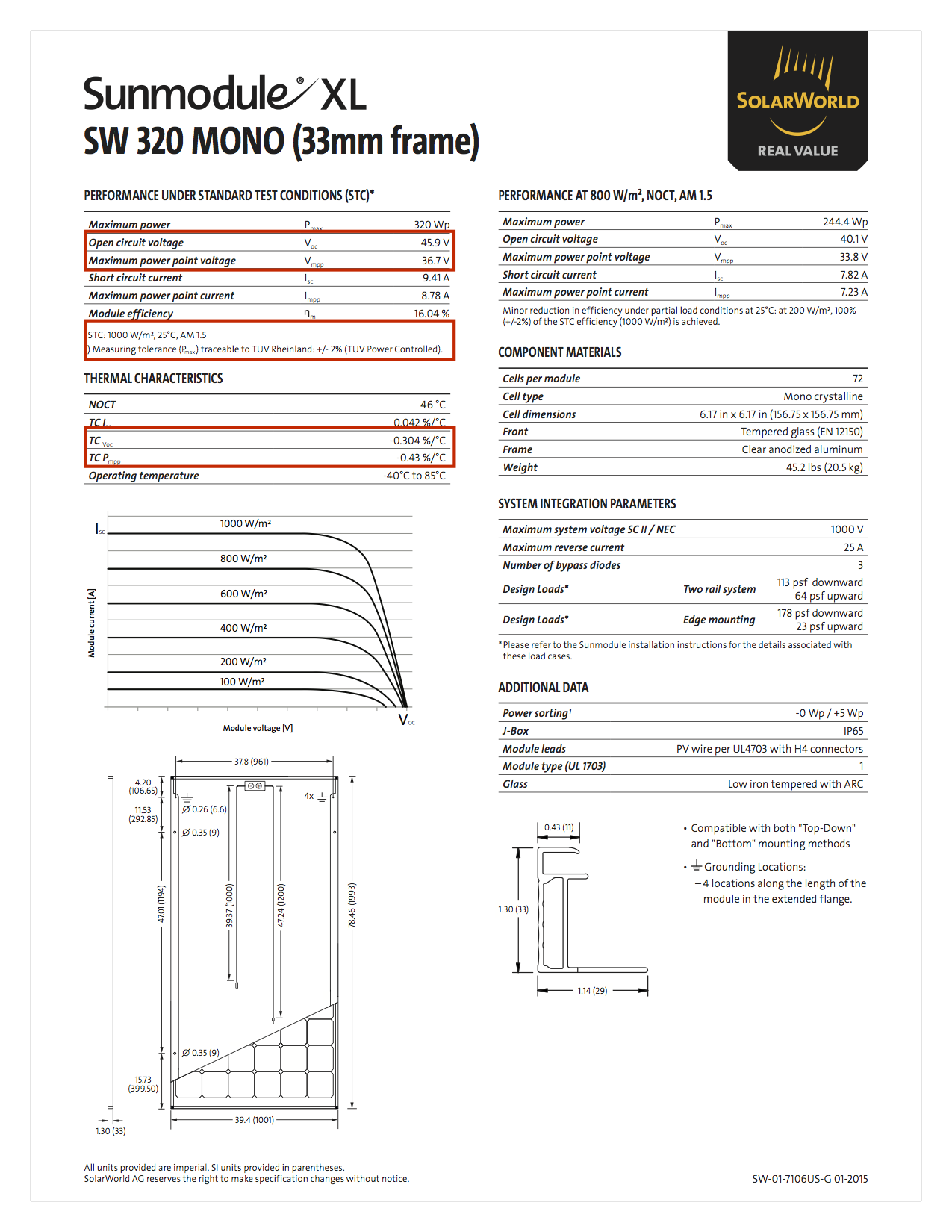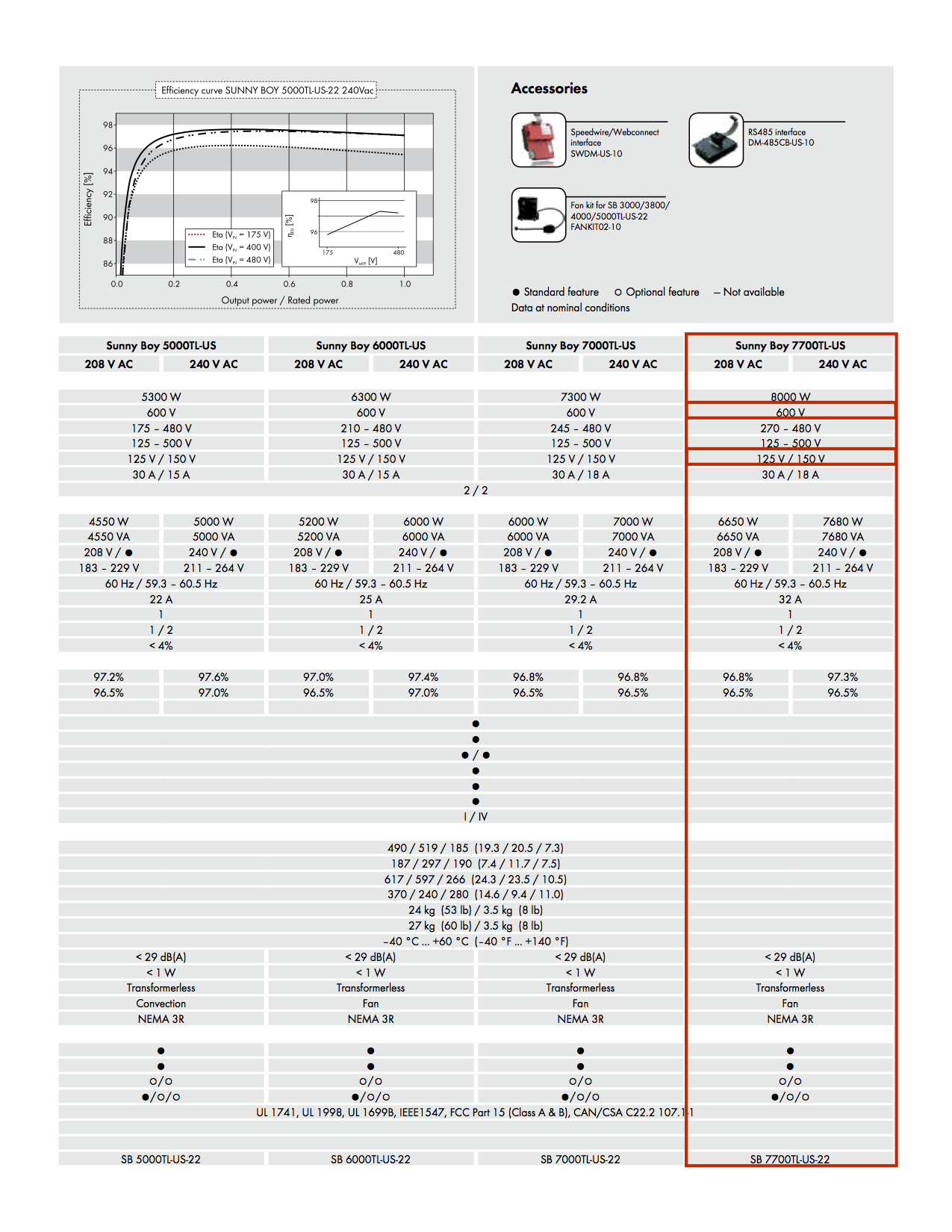# Solar Inverter String Design CalculationsFor many new to photovoltaic system design, determining the maximum number of modules per series string can seem straight forward, right?  Simply divide the inverter’s maximum system voltage rating by the open circuit voltage (Voc) of the module used and you’re good.  Well, that does get you in the ballpark, however, you could be at risk of over-sizing or under-sizing the number of modules in a string depending on where you are located in the world.  So in order to spot check ourselves, we can employ a couple of simple calculations to ensure that we do not encounter these set of problems.  There are a plethora of string-sizing tools out there.  Some are from the inverter manufacturers and others are intricate home-brew type tools.  These can be great to do the double checking for you, but often the module that we are using is too new, obsolete or simply not listed in the database of the tool that we are using.  Not to worry, these calculations are easy enough to do by hand or to input into a spreadsheet.Let’s just dive in, shall we?  I’ll set up a fictitious scenario with all the elements that we would need to be able to complete the calculations, including a module that is new enough that not many online string tools have it in their databases.

PV Module: SolarWorld Pro SW 320 XL Mono

The values that we need to collect from the datasheet is the Voc, cell temperature used for standard test conditions (STC),  temperature coefficient of Voc, maximum power point voltage (Vmp), and temperature coefficient of Vmp

Voc: 45.9, ºC @ STC: 25, TCVoc: -0.304, Vmpp: 36.7, TC Pmpp: -0.43Inverter Model:  SMA Sunny Boy 7700TL-US-22The two most important values to collect are the maximum DC input voltage and the start or strike voltage of the inverter.

600 Vmax, 150 Vstart

Now we need to dig up environmental data of the location.  There are two fantastic resources to use here and all you need to do is enter the zip-code of the location to find the weather data nearest to your location.  My next step is to go to SolarABC’s or SolarDesignTemps for the ASRAE data for Memphis, TN 38116.  I recommend the latter for areas outside North America for our international friends.

The important information to collect from either of these two sources are going to be a record low, the 2% annual design dry bulb temperature and the expected rise in cell temperature due to the mounting method (more on that here).

Let’s start by calculating the minimum number of modules that we should have in a series string.  This is a three-part calculation.

Vmin = (Vmp + ((THigh + TRise - TStc) x (VmpCoef x Vmp/100)))

Vmin = 36.7V + ((35ºC + 32ºC - 25ºC) x (-0.43 x 36.7/100)))

Vmin = 36.7 + (42 x -0.158)

Vmin = 30.064 V

Next we should factor in system loses (de-rate factor).  We forgo this calculation for the purpose of simplifying this exercise and assume our derate factor to be 12% or 0.88

Vmin = 30.064 x 0.88

Vmin = 26.46

We can now take our result from the above calculations to determine the bare minimum number of modules we can expect to have in our system by dividing our DC start (strike) voltage by our Vmin.

150 / 26.46 = 5.67 rounded up to the nearest whole number.

The minimum number of modules in series can be a low as 6.

Now we can calculate the maximum number of modules that we can have in our system by doing a very similar type of calculation.

Vmax = Voc + ((TLow - TStc) x (VocCoef x Voc/100))

Vmax = 45.9 + ((-12ºC - 25ºC) x (-0.304 x 45.9/100))

Vmax = 45.9 + (37 x 0.14)

Vmax = 51.08

Now, divide our result by the maximum DC system voltage of the chosen inverter and round down to the nearest whole number.

600 / 51.08 = 11.74

The maximum number of modules in series can be as much as 11.

Now we have all the parameters that we need to design a system which will not go over the maximum input voltage of the inverter at record lows and will meet the minimum start-up voltage of the inverter where cell temps are at their highest.

I like to keep a database of inverters and modules in a spreadsheet and populate the data needed in these calculations to save time, but you can simply plug these values into the formulas themselves if you are not too savvy with spreadsheets.

Tony commented 2 years ago

Where do I find Tlow?

Thanks

Now we need to dig up environmental data of the location.  There are two fantastic resources to use here and all you need to do is enter the zip code of the location to find the weather data nearest to your location.  My next step is to go to SolarABC’s or SolarDesignTemps for the ASRAE data for Memphis, TN 38116.  I recommend the latter for areas outside North America for our international friends.

TTQ commented 1 year 1 month ago

After that, what the best number of modules in each series can we choose?

Thank you for your comment. There are too many variables in the question to answer this with a generic answer.  The article describes how to calculate this, we recommend that the steps outlined are followed to find specific answers.  Feel free to contact us if you need additional engineering support. Thanks again.

Hassan Berbia commented 8 months 2 weeks ago

Thank you for your interesting Blog,
I am wondering for the case where the calculation lead to a the minimum number of strings is greater than the maximum.

Howard Johnson commented 5 months 3 weeks ago

Vmax = 45.9 + (37 x 0.14) is really Vmax = 45.9 + (-37 x -0.14)

Vmax = 45.9 + (-37 x -0.14) what the article is showing is correct since a negative multiplied by another negative is a positive. At the end of the day both are correct, the article shows a more simplified calc.

Kevin commented 5 months ago

How come your VMP is higher than VOC? I've never seen this and it's not possible.

Hello. Thank you for your comment. Looks like there is a misunderstanding.

This article shows Voc = 45.9V and adjusted for temperature to be 51.08V and shows Vmp = 36.7V and adjusted for temperature to be 30.064V.

Voc = 51.08V is greater than Vmp = 30.064V

Joseph commented 1 month 2 weeks ago

Is in it that Vmin w/ system loss (de-rate factor) should be:
Vmin = 30.064 / 0.88 ?

Thank you for your post. You need to multiply by the derate factor as shown in the article. Thanks.

Published
8 years 4 months ago
Written by